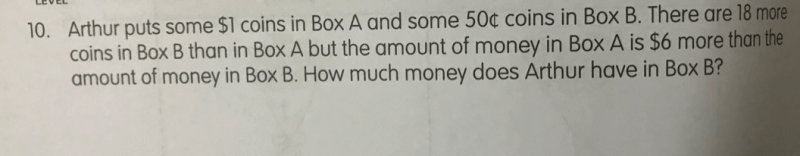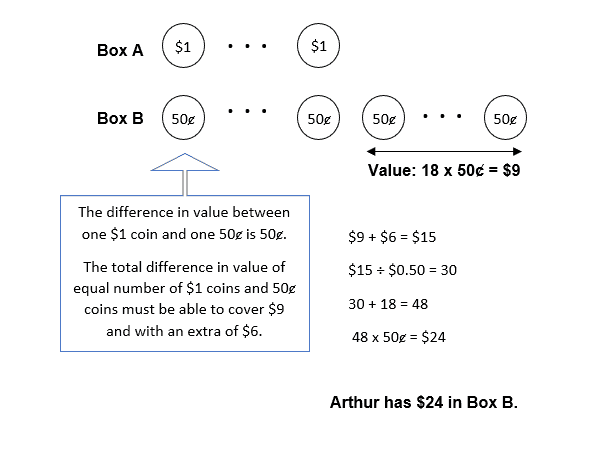# Questionappreciate anyone could help.

18 coins  x 0.5 =\$9

differences= 1-0.5=0.5

i understand that :

Box a :1 unit + \$9+\$6

box b: 1 unit +\$9

I m stuck. HTake note of number of coins vs value of coins.

The 1 unit you are referring to is the number of coins.

There are 1 unit of coins in Box A and (1 unit + 18) coins in Box B.